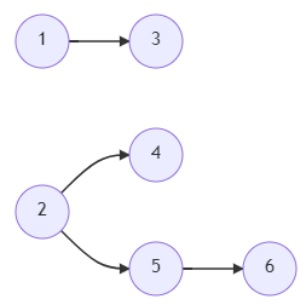# Program to count minimum semesters to cover all different courses in Python

PythonServer Side ProgrammingProgramming

Suppose we have a number n, indicates that there are n different courses labeled from 1 to n. We also have an array called relations where relations[i] contains a pair (prevCourse_i, nextCourse_i), representing a prerequisite relationship between the course prevCourse_i and the course nextCourse_i: so course prevCourse_i has to be taken before the course nextCourse_i. And the last parameter we have that is k. In one semester, we can take at most k number of courses as long as we have taken all the prerequisites in the previous semester for the courses we are taking. We have to find the minimum number of semesters needed to take all courses.

So, if the input is likethen the output will be 3 because in the first semester we can take course 1 and 2, now we are eligible for course 3, 4 and 5, so if we take 5 and any one of 3 or 4 for the second semester, then we can end all courses in the next semester. So we need 3 semesters in total

To solve this, we will follow these steps −

• taken := a new set

• g1 := a list with n empty lists

• g2 := a new list of size n and fill with 0

• w := a new list of size n and fill with 0

• semester := 0

• for each x in relations, do

• insert x-1 at the end of g1[x-1]

• insert x-1 at the end of g2[x-1]

• weight := a new list from the length of all items in g1

• for i in range 0 to n - 1, do

• for each x in g1[i], do

• w[x] := maximum of w[x] and weight[i]

• while size of taken < n, do

• courses := a new list

• for i in range 0 to n - 1, do

• if g1[i] is empty and i is not in taken, then

• insert (i, w[i]) at the end of courses

• if size of courses > k, then

• courses = sort courses based on the second parameter in reverse order

• courses := list of first k courses

• semester := semester + 1

• for each x in courses, do

• for each y in g2[x], do

• delete x from g1[y]

• g2[x] := empty list

• insert x into taken

• return semester

## Example

Let us see the following implementation to get better understanding

def solve(n, relations, k):
taken = set()
g1 = [[] for _ in range(n)]
g2 = [[] for _ in range(n)]
w =  * n
semester = 0
for x in relations:
g1[x-1].append(x-1)
g2[x-1].append(x-1)

weight = list(map(len, g1))
for i in range(n):
for x in g1[i]:
w[x] = max(w[x], weight[i])

while len(taken) < n:
courses = []
for i in range(n):
if (not g1[i]) and (i not in taken):
courses.append([i,w[i]])
if len(courses) > k:
courses = sorted(courses, key = lambda x:x,reverse=True)
courses = courses[:k]

semester += 1

for x in courses:
for y in g2[x]:
g1[y].remove(x)
g2[x] = []
return semester

n = 6
relations = [(1,3),(2,5),(2,4),(5,6)]
k = 2
print(solve(n, relations, k))

## Input

6, [(1,3),(2,5),(2,4),(5,6)], 2


## Output

3